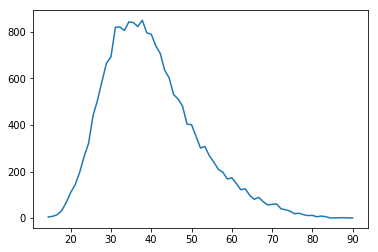# A Mutable Log

A blog by Devendra Tewari

Project maintained by tewarid Hosted on GitHub Pages — Theme by mattgraham

# Using Python to analyze data in a PDF file

The state university my daughter wants to study at just announced their entrance exam results via a PDF file. I wanted to get additional insights from the data, and decided it was time to use Python—I’ve got Jupyter Notebook installed on macOS—to do the data extraction and analysis.

I needed to install a few additional packages for python 3

pip3 install pdftotext pandas matplotlib


First, I created an empty DataFrame with the three columns I needed

import pandas as pd
columns = ['id','name', 'result']
df = pd.DataFrame(columns=columns)


Next, I read text data from the PDF

import pdftotext
with open('SSA1_2018_Publicacao_v4.pdf', 'rb') as f:
pdf = pdftotext.PDF(f)
print(len(pdf))


Next, I parsed the text data into the DataFrame

k = 0
for i in range(len(pdf)):
lines = pdf[i].split('\n')
for j in range(3, len(lines) - 3, 1):
words = lines[j].split()
idn = words
name = ' '.join(words[1:-1])
if not name:
continue
result = float(words[-1].replace(",", "."))
if result == 0.0:
continue
df.loc[k] = [idn, name, result]
k = k + 1
print(k)


Next, I sorted the DataFrame by the result column to find the top scorers

df=df.sort_values(by=['result', 'name'])
print(df.tail())


Next, I grouped students by their score to find how many had the same score as my daughter’s

df_grouped = df.groupby(by='result')['result'].count()
print(df_grouped)


Finally, I plotted the grouped data

%matplotlib inline
import matplotlib.pyplot as plt
plt.plot(df_grouped)